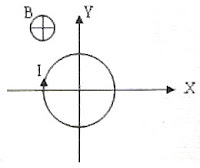## Tuesday, April 10, 2007

### MCQ involving Magnetic Force on Current carrying ConductorsQuestions on magnetic force on current carrying conductors are interesting and often simple. Occasionally you may find questions which may baffle you. See the following question:

A copper wire is bent in the form of a sine wave of wave length ‘λ’ andpeak to peak value ‘a’ as shown in figure. A magnetic field of flux density ‘B’ tesla acts perpendicular to the plane of the figure in the entire region. If the wire carries a steady current ‘I’ ampere, the magnetic force on the wire is

(a) I√(a22) B (b) IaB (c) I(a+λ)B (d) I(λ– a)B (e) IλB

This is a very simple question and the correct option is (e).This is a very simple question and the correct option is (e).

Imagine the wire to be made of a large number of horizontal and vertical elements as shown. There are as many vertical elements carrying current upwards as there are those carrying currents downwards. The magnetic forces on them will be leftwards and rightwards and they will get canceled. But the magnetic forces on the horizontal elements will be in the same direction ( either upwards or downwards, depending in the directions of the magnetic field and the current) and they will get added to produce a net force IλB.

The following MCQ appeared in IIT–JEE Screening 2003 question paper:

A conducting loop carrying a current ‘I’ is placed in a uniform magnetic field pointing in to the plane of the paper as shown. The loop will have a tendency to(a) contract

(b) expand

(c) move towards positive X-axis

(d) move towards negative X-axis

The magnetic force everywhere on the loop is radially outwards as given by Fleming’s left hand rule. So, the loop has a tendency to expand [Option (b)].

In the above question, suppose that in place of the option ‘expand’, you had the option, ‘move towards the positive Z-direction’. In that case also, the correct option would be (b) because the loop will act as a magnetic dipole whose south pole is the nearer face. The loop will therefore move towards the reader.